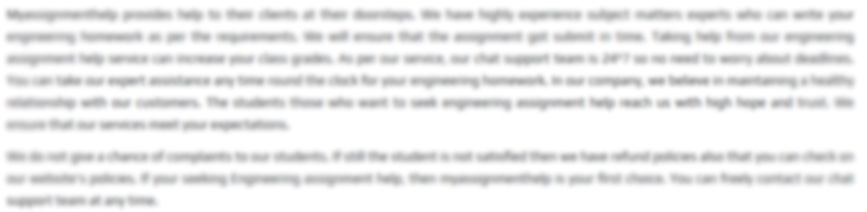Call Back

#### ENGIN5202 - Advanced Structural Analysis and Design

Question Preview

ENGIN5202 Advanced Structural Analysis and Design Assignment 2 Design of a R.C Column and a PSC Beam THE STRUCTURE The attached diagram depicts a proposed detail in one area of a large shopping complex and associated carpark in an Eastern suburb of Melbourne (more than 1 km from the coast). The reinforced concrete h one side, a prestressed concrete box girder for a single-lane ramp into the carpark and, on the other, a large beam which, in turn, supports other structural members which are part of the retail area. The proposed cross-sectional dimensions of the box girder ramp are shown. The ramp is simply supported on rubber bearings which are seated on the short cantilever beam cast into the side of the column. The ramp actually comprises a number of individual spa L L The beam on the othe

DESIGN REQUIREMENTS

a)     Design of the R.C. Column

Design a suitable cross-section (size and reinforcement longitudinal and ligatures) for this column and summarize your design on a simple sketch.

AS3600

Rectangular column – b = 0.75% D

Bars on two sides = 3.5% As

Height = 7m

Wr = 17 KN/m

G = 1050 KN

Q = 580 KN

Density = 21.5 KN/m^2

2. Ramp on LHS and beam on RHS (DL only)
3. Ramp on LHS (DL) & beam on RHS (DL only)
4. Ramp on RHS (DL + LL) & Beam on RHS (DL)
5. Ramp on LHS (DL + LL) & Beam on RHS (DL + LL)

Second order effect to be considered

Derive strain inter action diagram

Self weight of ramp = 1.236*245 = 38.282 KN/m

DL of Ramp = 17 KN/m

LL = 391 KN

Assuming size of column = 750*1000 mm

B = 750 & D = 1000 mm

Le = 0.85*7 = 5.95 m

r = 0.3*1000 = 300 mm

Slenderness ratio = Le/r = 5950/300 = 19.5 < 25

Column is short

Fc’ = 32 MpaFy = 500 Mpa

Case 2:

N = 1050 + 697 = 1747 KN

M = 1030*0.75 – 697*1.8 = 467 KNm

Case 3:

N = 697 + 1050 + 580 = 2327 KN

M = 697*1.8 &ndGet solution

#### \$ 30.00

Orginal Price : \$ 40.00